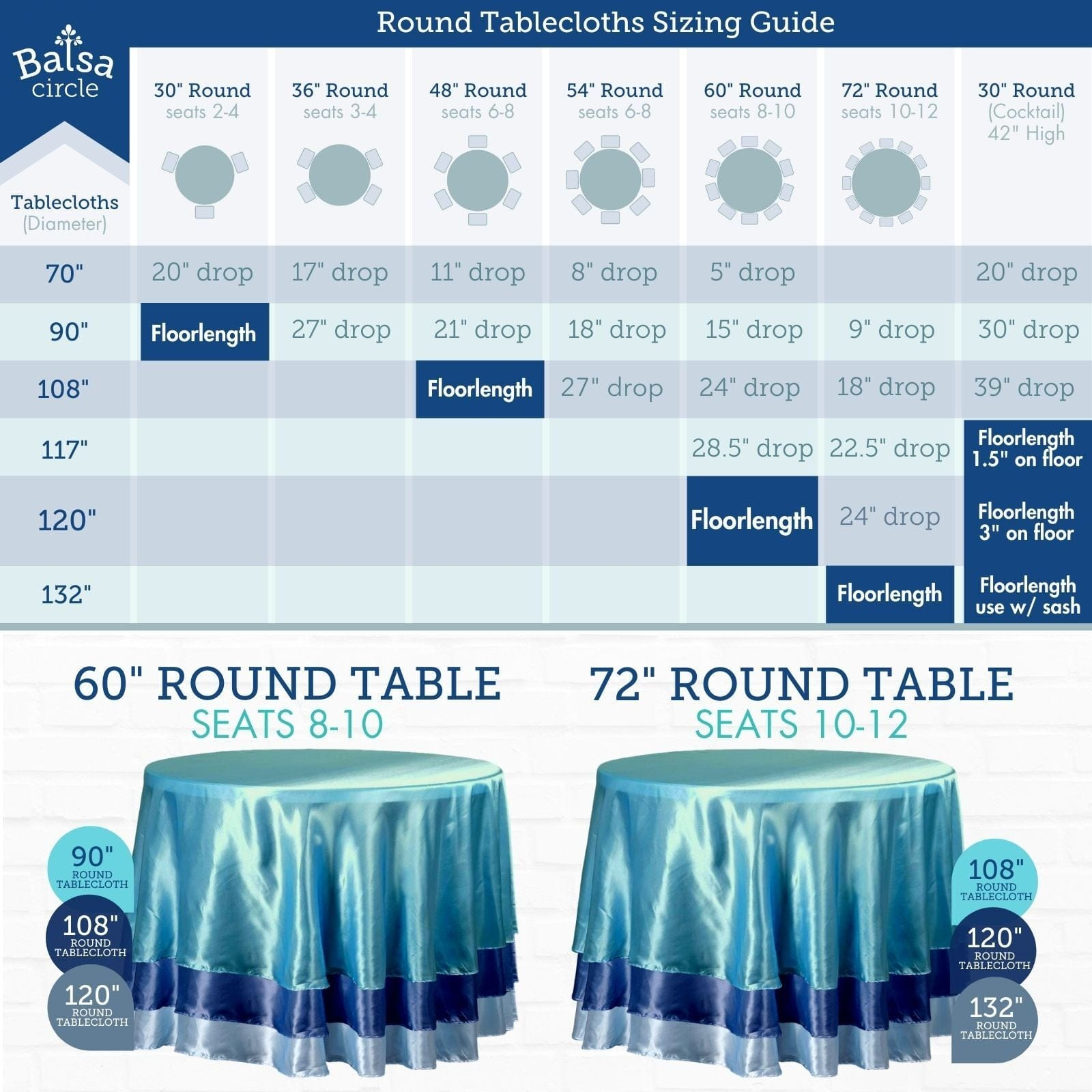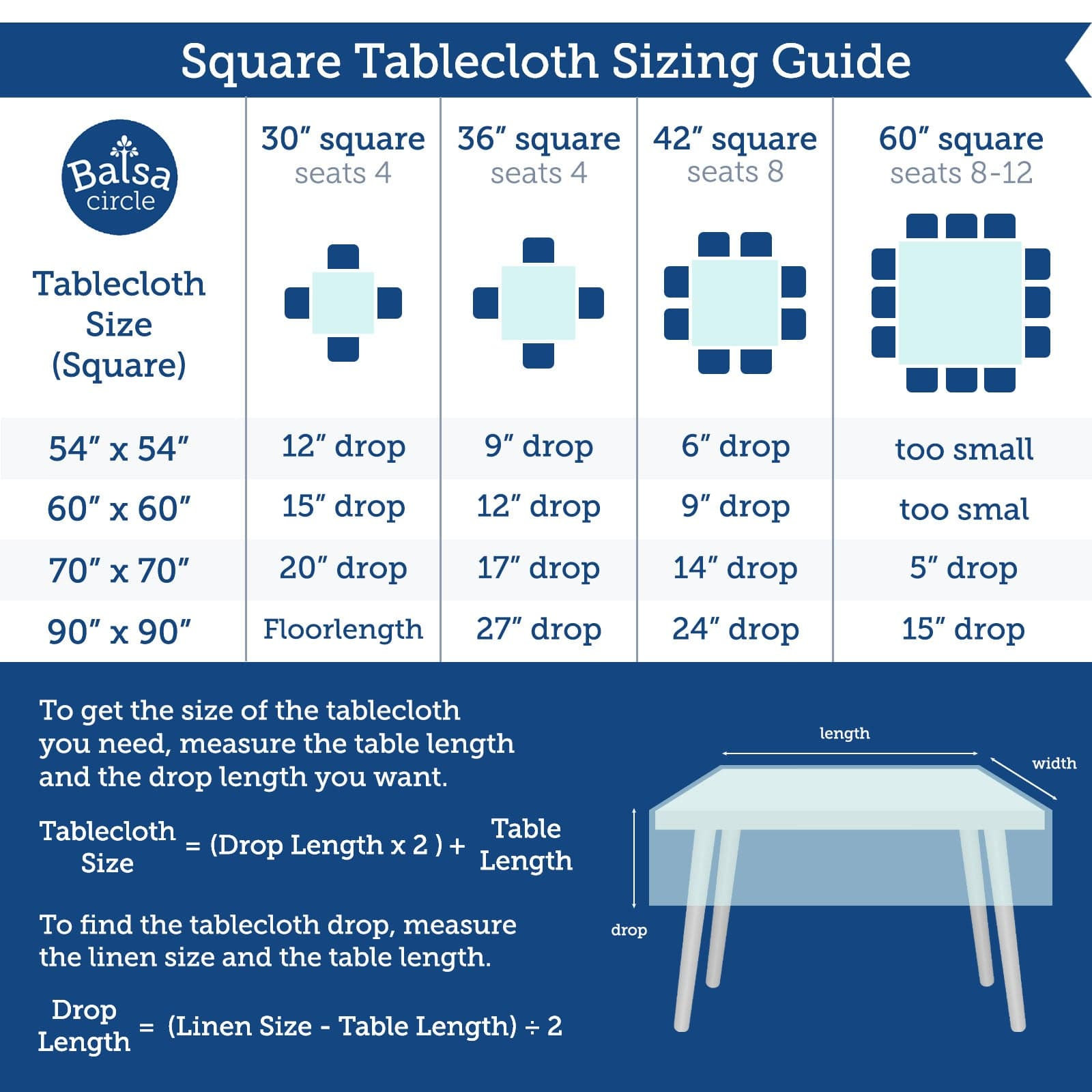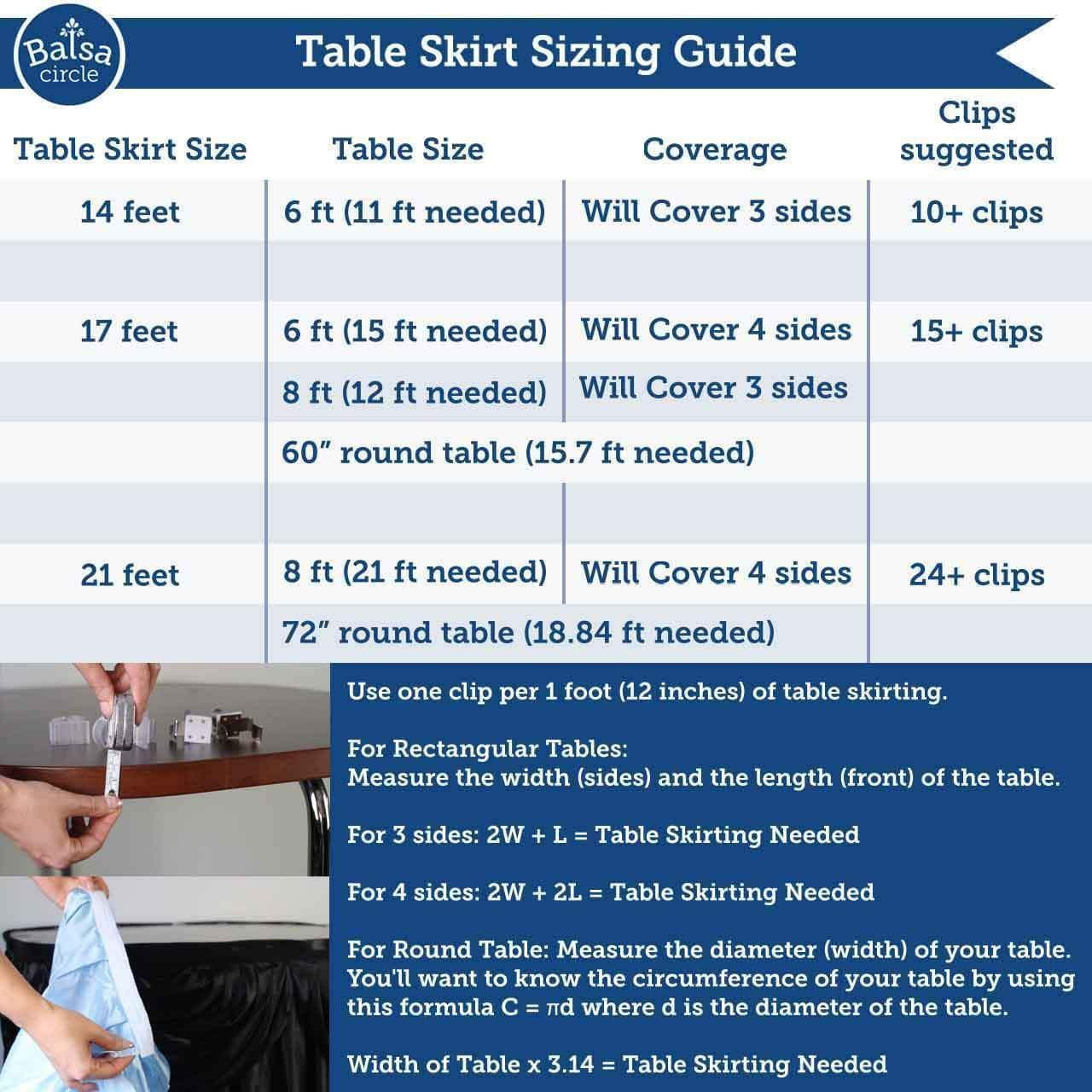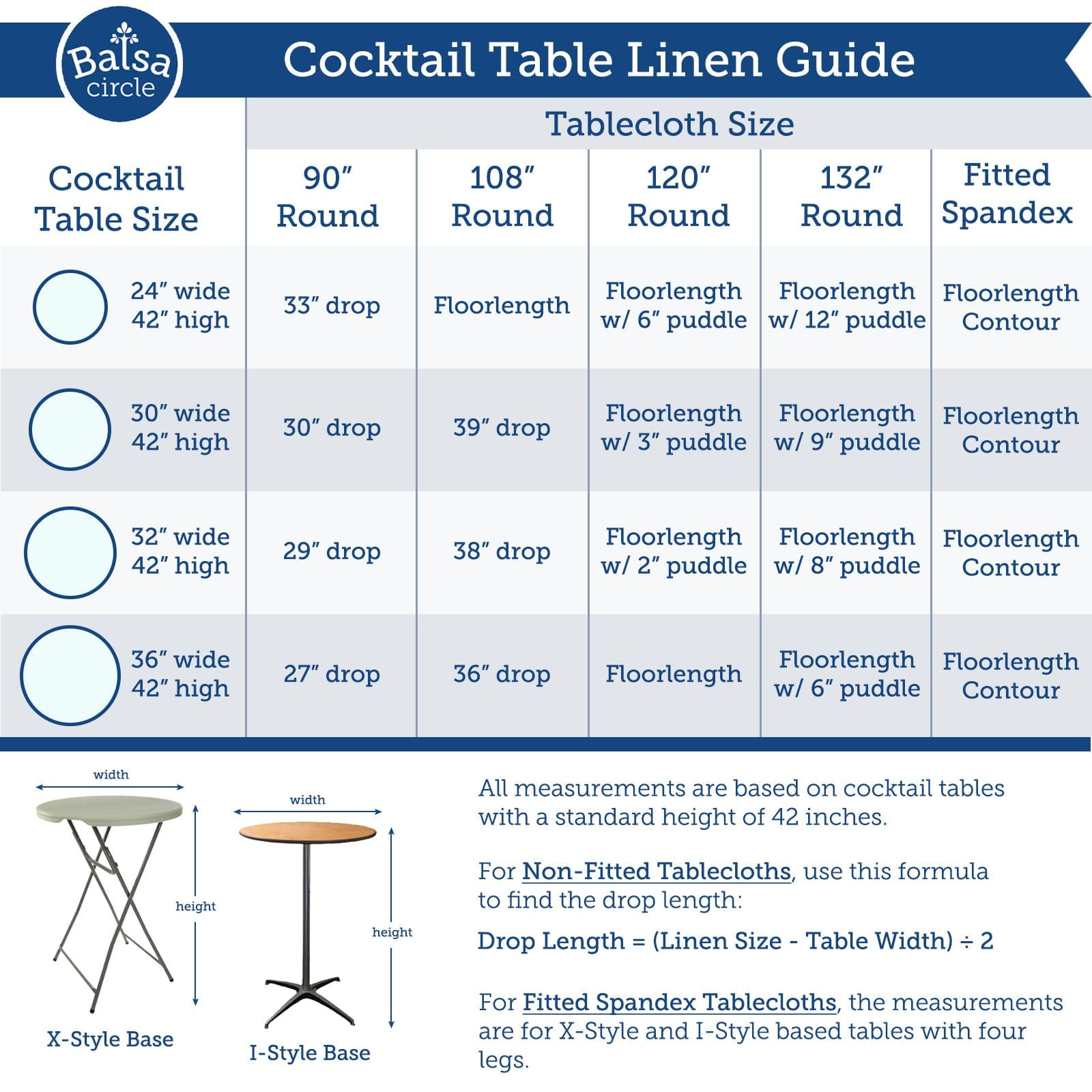# Tablecloths and Linens Sizing Guides

To find the right tablecloth, make sure you know the table size or measure your event tables before purchasing.

Round Tables

Measure your table diameter (or radius x 2) then add the desired drop x 2. If you want the tablecloth to go to the floor on standard size tables (or drop 30” to the floor) add 60, if you want it to go half way (or drop 15” to the floor) then add 30.

Diameter of your table + (Drop x 2) = Tablecloth size

If you’re not sure how long the tablecloth drop is, then subtract the diameter of the table from the tablecloth size and then divide by 2.

(Tablecloth size - Diameter of your table) / 2 = Drop

For example, your table is 60” round and you want the tablecloth to go to the floor, or have a 30" drop to the floor. Simply multiply 30 x 2 = 60” and add 60” to the diameter of your table (60 + 60 = 120”) so the tablecloth should be 120” round.Overlay Sizing

You can use square overlays for most tables or smaller round tablecloths for round tables. To find the right overlay, make sure you know your table size (width, length and height) so we recommend that you measure your tables before purchasing linens. For more overlay sizing help, visit our blog

Here's the formula for overlays to use with a round table -

(Overlay Length - Your Table Diameter) / 2 = Overlay Drop

If you are measuring for a rectangular table, the formula is similar -

(Overlay Length - Your Table Length) / 2 = Overlay DropSquare Tables

If you know your table size, simply multiply the desired drop length by 2 and then add the table length. If you’re not sure how long the tablecloth will drop, subtract the table size from the linen size and divide by 2.Rectangular Tables

First, measure the width, height and length of your table. The height of the table would equal the drop if you’d like the tablecloth to go all the way to the floor. Once you have measured the table, add twice the desired drop to the length and the width since the drop is on both sides of the table.

D x 2 = D where D is the drop of the table.
2D + Length of your table = Length of tablecloth
2D + Width of your table = Width of the tablecloth

If you’re not sure what the drop should be, use these formulas:

(Length of tablecloth - length of table) / 2 = Drop of tablecloth lengthwise
(Width of tablecloth - width of table) / 2 = Drop of tablecloth on wide side

For example, your table is 30" x 72". If you want a 30" drop to the floor, multiply 30 x 2 = 60” and add 60” to your width (30 + 60” = 90") and length (60 + 17 = 132"), so the tablecloth should be 90" x 132".Table Skirts and Clips Sizing Guide

First, measure the width (sides) and length of your rectangular table or the diameter if you're using a round table.

For table clips, you’ll want to measure the width of your table edge first. Then choose the table clip from the shop based on the width of your table edge. Use one clip per 1 foot (12 inches) of table skirting. The outside of the clip will have a velcro strip that you'll use to attach the table skirt to the table.

If you have a rectangular table: For the table skirt, you'll want to decide if you want to skirt 3 sides or all 4 sides. To find out how many feet of skirting you'll need to know the measurements of your table, the width and the length.

For 3 sides, use this formula : 2W + L = Table Skirting Needed

For 4 sides, use this formula : 2W + 2L = Table Skirting Needed

For example, if we have a 6 ft table, that's 2(30) + 72 = 132 inches so you'll need to cover 132" or 11 feet. In

If you have a round table: You'll want to know the circumference of your table by using this formula C = πd where d is the diameter of the table.

Width of Table x 3.14 = Table Skirting NeededCocktail Table Linen Guide

All measurements in this guide are based on standard cocktail tables or high-boy tables with a height of 42 inches.

If you are using a Non-Fitted Round Tablecloth, measure the size of your linen and the diameter or width of your table. Find the drop length using this formula:

Drop Length = (Linen Size - Table Width) / 2

Our Fitted Spandex Cocktail Tablecloths comes with leg pockets and are ideal for X-style base and I-style base cocktail tables with four (4) legs.